>Dr. J's Maths.com
Where the techniques of Maths
are explained in simple terms.

Applications of Calculus - Natural growth and decay.
Test Yourself 1.

REMEMBER: The natural growth and decay models are based on the concept that

the rate of change of a quantity varies directly with the size/amount of that quantity at any instant.

 The questions on this page focus on: Growth - direct statement; Growth - statement of the derivative; Decay - direct statement; Decay - statement of the derivative.

 Growth Direct statement 1. The number of bacteria (P) in a colony after t minutes is given by P = 1000 e0.04t. Find: (i) the number of bacteria (to the nearest 10) when t = 10. (ii) the rate at which the colony is increasing when t = 10. (iii) how many minutes it takes for the colony to double in size from its original number. Answer.(i) 1490 bacteria. (ii) increase is about 60 bacteria/min.(iv) 17 mins 20 secs. 2. At the beginning of 2015, there were 1,200 people in the population of a small country town. A number of renewable energy companies were establishing sites in the town and it was expected that the population of the town would increase according to the model N(t) = 1200 ekt where t is the number of years and k is a constant. At the beginning of 2019, the population had grown to 1500 people (to nearest 100). (i) Show that k = 0.0558 (correct to 4 decimal places). (ii) Find in years and months, correct to the nearest month, the time it will take for the population of the town to increase to 2,000. (iii) At what rate was the town growing in that year compared to the rate in 2015? Answer.(ii) 9 years 2 months.(iii) increase in 2024 will be about 112 people per year compared to about 67 per year in 2015. 3.The populations of two mining towns - town A and town B - have been growing during the iron ore resources boom. Their respective populations (ii) M0 = 100 k = 1.15 × 10-4. (iii) mass is about 63 grams. (iv) 26,000 years.N years after 1 January 2014 can be expressed as Town A: NA = 2000 e0.13t Town B: NB = 1500 e0.18t (i) Find, to 2 significant figures, the population of town A at the start of 2019. (ii) Find, in which year, town B's population doubled. (iii) In which year and month did town B's population pass town A's population? Answer.(i) 3,800 people. (ii) In 2017. (iii) October 2019. 4. The diameter of a tree (D cm) t years after the start of a particular growth period is given by D = 80ekt (i) Show that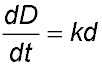where k is a constant. (ii) If k = 0.0018, how long (to the nearest year) will it take for the diameter of the tree to measure 90 cm. Answer.(ii) 65 years. 5. In a particular native bush reserve (not too far away) the population of (unwanted) rabbits can be determined by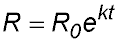where R0 and k are constants. When the first count of the rabbits was made, there were 200. After only 5 weeks, the number had increased to 440 (gestation period is about 27 days). (i) Determine the values of R0 and k. (ii) Calculate the number of rabbits in the reserve after 20 weeks (to the nearest 10 rabbits). (iii) How long (in days) will it take for the number of rabbits to reach 6,000? (iv) How many rabbits per week were being added to the population after 20 weeks? Answer.(i) R0 = 200; k = 0.1577. (ii) 4,690 (pests). (iii) 151 days. (iv) 740 being added per week. 6. The population of butterflies in a rain forest is (thankfully) growing at an exponential rate according to the model N = 300 ekt where t is the time in months after the butterflies were first counted. At the end of three months the number of butterflies in that area had doubled. (i) Calculate the value of the constant k (4 decimal places). (ii) How many butterflies should there be after 12 months? (to the nearest 100) (iii) At what rate is the butterfly population growing after three months? (to the nearest 10/month). (iv) If the number of butterflies after 12 months was 5,200, what comment could you make on the model? Answers.(i) k = 0.2310.(ii) 4,800 butterflies. (iii) 140 butterflies per month.(iv) There are more butterflies than expected so perhaps the value of k has increased (which would be excellent). Statement of derivative 7. The rate at which Cameron's investment V is growing is proportional to the value of the investment - so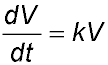. (express all money answers to nearest \$100). (i) Show that V = V0ekt satisfies the equation. (ii) Ten years ago, Cameron purchased a block of land for \$120,000. It is estimated that the land will be worth \$1,300,000 in another 15 years time. Use this information to find the values of V0 and k (express k to 4 decimal places). (iii) At what rate is the value of the property increasing today (i.e. 10 years after Cameron purchased it) to the nearest \$100 per year? (iv) In 5 years time, Cameron is expecting to be offered \$290,000 to sell the property. Would he be making a good financial decision if he accepted that offer in 5 years? (v) If he rejected the "5 years into the future" offer and, in 8 years time from now, the value of his property was actually \$595,000, what conclusion can you reach about the model? Answer.(ii) V0 = 120,000 and k = 0.0953. (iii) Rate = 0.0953 × 311,217 = 29,700 per year. (iv) Predicted value is \$311,217 so maybe better to not sell. (v) the model does not still apply as the value of k has decreased (to 0.089) and the predicted value is less than the \$667,000 predicted by the original model. 8. The number, N, of bacteria in a culture grows at an hourly rate given by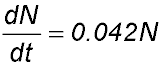. When first placed into the dish, there were 1 million bacteria. (i) How many bacteria are present after 20 hours? (ii) How long did it take for the number in the culture to double? (iii) What is the percentage rate of increase of the population? (iv) If the temperature of the culture is changed, it is found that the rate (per hour) of growth is reduced to 2.1% of the presnt amount. Write a new equation for the number of bacteria based on the increased temperature. (v) Draw a suitable sketch to show the difference between the two equations. Answer.(i) At 20 hours, N = 2.316 × 106. (ii) 16.5 hours. (iii) 4.2% of the number present. (iv) N = 106e 0.021t. 9. The present temperature of a star is 8000° C and it is losing heat continuously. The rate of heat loss is defined by the equation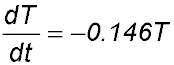where T is the temperature in degrees Celsius and t represents time in millions of years. Find the star's temperature in 10 million years. Answer. 1858°. 10. The bacteria on cheese grow in direct proportion to their number B at any given time t. (i) Write down this statement as a rate equation. (ii) The initial bacterial count was 1000 and one hour later, the count had risen to 1200. Find a value for the growth constant k. (iii) How many bacteria were on the cheese after 2 hours? (iv) At what rate were they increasing after 2 hours? (v) The cheese is regarded as being unsafe when the number of bacteria is three times the original count. What is the maximum time for the cheese to be left out if we impose a 10% buffer on the count (to be sure)? Answer.(ii) k = 0.1823.(iii) 1,440 bacteria.(iv) 162 bacteria/hour.(v) 5 hours 27 minutes. Decay Direct statement 11. The half-life of radium-226 is 1390 years. This means that the rate of decay is proportional to the amount present and half of any given quantity will decay in 1390 years. The rate at which radium-226 decays is given by M = M0ekt where M is measured in milligrams and t in years. (i) Use the information above to find the value of k. (ii) After how many years will 20% of the initial quantity remain? Answer.(i) k = -0.0005..(ii) 3,219 years. 12. The population in a country mining town was recorded at the start of 2010. The population P - t years later - is described by the exponential equation P = 120,000 e -0.05t. (i) What was the initial population of the town at the start of 2010? (ii) Find the time, in years and months, it will take for the population to halve. (iii) At what rate is the population changing at the start of 2019? 13. The mass M grams of a radioactive isotope of carbon found in a rock sample at time t is described by the equation M = M0 e-kt where M0 and k are constants. (i) Prove that the rate of decay of the mass of the carbon isotope is proportional to the mass present at any time t. (ii) If there is initially 100 grams of the isotope and this mass decays to 75 grams in 2,500 years, find the values of M0 and k. Give your value of k correct to 3 significant figures. (iii) Find the amount of the isotope present at the end of 4,000 years. Express your answer to the nearest gram. (iv) Find the time required for the mass of the isotope to decay to 5 grams. Express your answer correct to the nearest 100 years. Answer. 14. Ten kilograms of sugar are placed in a container of water and begin to dissolve. After t hours, the amount of undissolved sugar is given by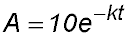(i) Calculate the value of k given that A = 3.2 when t = 4. (ii) After how many hours does 1 kg of sugar remain undissolved? Answer. 15. When the voltage is switched off in a television set, the current I amps flowing in the circuit decreases with time t seconds as modelled by the equation I = 0.5e-5t. (i) What is the current flowing through the circuit after 0.25 seconds? (ii) How long will it take for the current to be reduced by half? Answer.(i) 0.1433 amps(ii) 0.1386 seconds. Statement of the derivative. 16. A block of ice is removed from the refrigerator. The rate at which the ice melts is proportional to the amount remaining - i.e.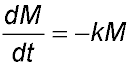where M is measured in grams and time in minutes. (i) Show that M = M0e-kt is a solution of the equation. (ii) After 35 minutes, only half of the ice remains. Find the value of k to 3 significant figures. (iii) If, at a certain time, only 5% of the block of ice remains, how long would it have been since the block was removed from the refrigerator (answer in hours and minutes). 17. A metal ball is fired into a tank filled with a thick viscous liquid. The rate of decrease of its velocity is proportional to its velocity cm/second. Therefore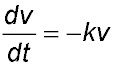where k = 0.07 and t is the time in seconds.The initial velocity of the ball when it enters the liquid is 85 cm/second. (i) Show that v = 85 e -0.07t satisfies the equation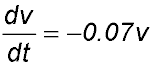(ii) Calculate the velocity and the acceleration when t = 5 (to 3 significant figs). Answer.V = 60 cm/sec. acc = 18. A radioactive substance decays at a rate proportional to the amount present. After two years, 10% of the original amount has decayed. What is the half-life of the substance (i.e. the time for 50% of the substance to have decayed)? Answer.13.15 years. 19. After its engine is cut, a biplane travels in a straight line with negative acceleration proportional to its velocity - that is. The plane is travelling at 400 m/sec when the engine is cut and 10 seconds later the velocity is 250 m/sec. (i) Show that this equation is satisfied by the equation v = v0 e-kt. (ii) Find the value of v0 and k. (iii) Find how long it takes for the velocity to fall to 100 m/sec (to the nearest m/sec). 20. A quantity Q of a radioactive substance decays at a rate that at any time t is proportional to the amount remaining at that time. That is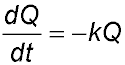where k is a positive constant. Q0 is the amount of the substance at t = 0.   At t = T, the quantity of the substance has deceased to Q1. (i) Show that Q = Q0e-kt is a solution of the differential equation. (ii) Express the value of the constant k in terms of T, Q0 and Q1. (iii) Show that the amount of the radioactive substance remaining at time nT can be expressed as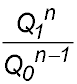.

.